## What is Sinusoidal Pulse Width Modulation?:

In Sinusoidal Pulse Width Modulation the pulse-width instead of being uniform as in the waveform of Fig. 11.55 is a sinusoidal function of its angular position with respect to a reference sine wave resulting in a reduction in the harmonic content. The control function consists of a sinusoidal wave obtained from an oscillator of variable amplitude A and of fundamental output inverter frequency f = 1/T as well as a triangular wave of fixed amplitude Ap and frequency fp with a direct component of amplitude Ap as shown in Fig. 11.56.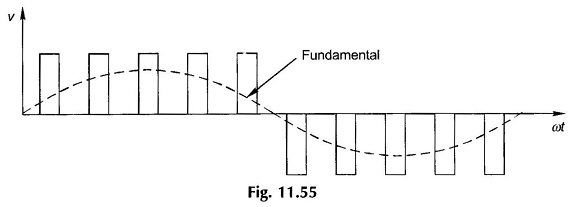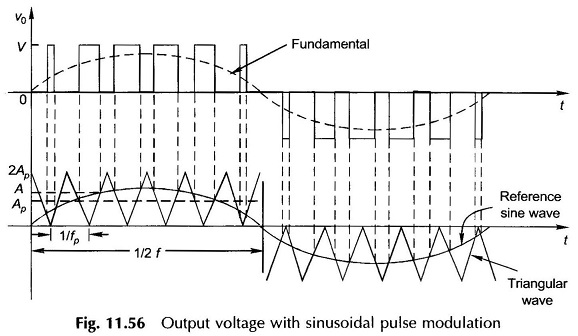The biased triangular waveform is reversed in polarity at the end of each half-cycle of the output voltage. Pulsed gating signals are generated by comparison of the sinusoidal and triangular waveforms—gating pulses are obtained for time intervals in which the sinusoidal signal is more positive than the triangular signal.

It is easily established from the figure that the number of gate pulses (sinusoidally modulated) per half-cycle is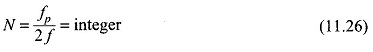It follows from the above that the angles for turn-on and commutation of the thyristors are determined by the intersections of the two above referred signals. It can be easily seen from Fig. 11.56 that the amplitude of the fundamental voltage can be controlled by varying amplitude A of the sine wave over the range 0 < A < Amax where Amax = 2AP. If A is made larger than Amax, the number of output pulses becomes less than N (Eq. (11.26)). In the limit the output becomes a single rectangular pulse of half-cycle width as in the case of ordinary (unmodulated) bridge inverter.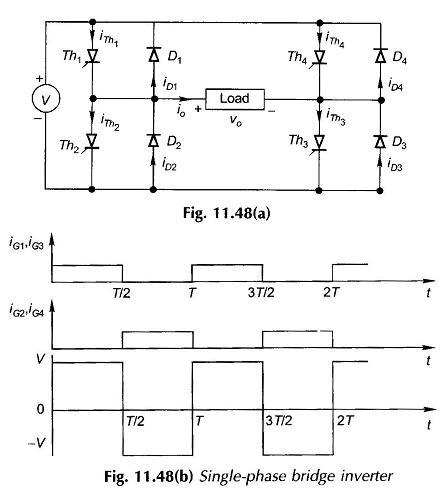The gating signals obtained as the above are employed to fire the thyristors in the bridge inverter of Fig. 11.48. Thyristors Th1 and Th3 are gated during the interval of a positive pulse and Th2 and Th4 are gated during the negative pulse. During the zero-value intervals of the pulsed-gating signals Th1 and Th4 or Th2 and Th3 are gated resulting in zero output voltage. Thus the output voltage as shown in Fig. 11.56 is a replica of the pulsed-gating signals generated by comparison of sinusoidal and triangular waveforms fed to the commutating circuitry.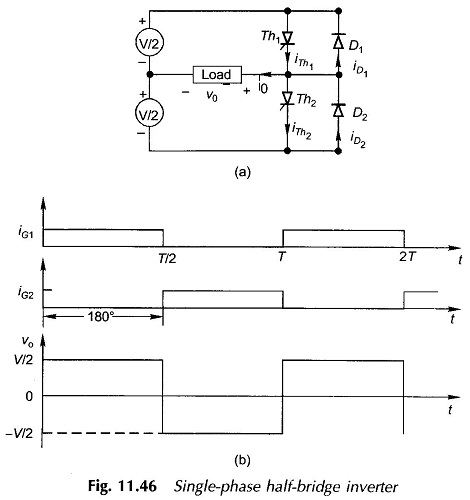Examination of the circuit of the half-bridge inverter of Fig. 11.46(a) reveals that there is no way in which the load voltage can be reduced to zero. At all times, ν0=±Vdc/2. The above method of Sinusoidal Pulse Width Modulation, therefore, cannot be applied as such. A modified method of modulation that can be employed in the half-bridge inverter is illustrated in Fig. 11.57. Voltage control is achieved by varying the amplitude of the reference sine wave. This type of voltage control is rarely employed because of its high ratio of harmonic amplitudes to the amplitude of the fundamental component of the output voltage wave.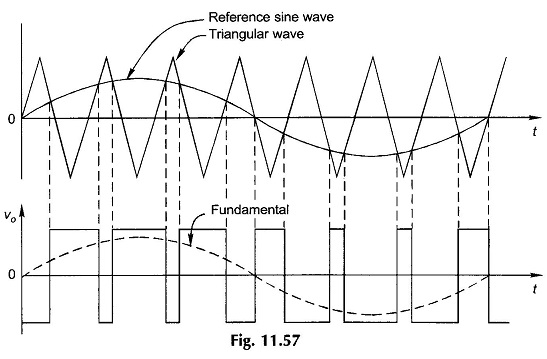The use of a pulse-width modulated (PWM) inverter for ac motor control is illustrated in Fig. 11.54 where the inverter is fed from a substantially constant dc voltage diode rectifier, while the control of ac voltage at motor terminals is achieved through the inverter.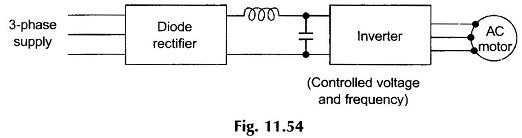The chief advantage of this scheme of control lies in the fact that it requires a single controllable power conversion so that the power factor of ac supply to the diode rectifier is high. Further, the low-frequency harmonic content of the PWM inverter is lower than that of an ordinary (unmodulated inverter). These advantages of the PWM drive are obtained at the cost of greater sophistication in the control logic. In PWM drive the inverter efficiency is somewhat decreased because of many commutations per half-cycle. The commutation frequency should be increased as permitted by the devices so as to obtain a good balance between increase in inverter loss and deem’ se of machine loss (due to reduced harmonic content).

Of late, the technique of selected harmonic elimination in PWM inverters has received wide attention. In this method, notches are created at predetermined angles of the square wave which permits voltage control with elimination of selected harmonics.

Scroll to Top